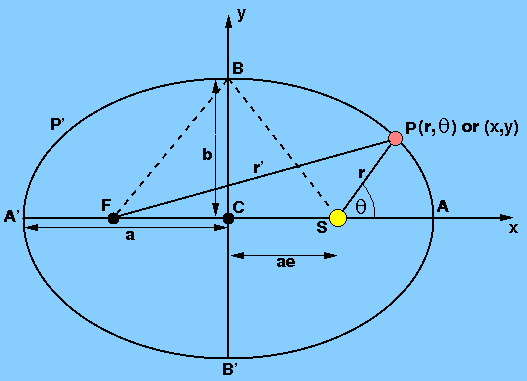Using the planetary distance determination method that he derived in conjunction with the positional observations of the planets that Tycho Brahe had painstakingly accumulated over 20 years, Kepler set about tracing the orbit of Mars. He soon found that the age-old idea of circular orbits had to be discarded, as the distance of Mars from the Sun appeared to vary. Kepler tested many models of orbital shapes and finally showed that the orbital planes of the planets pass through the Sun and that the orbital shape was an ellipse. These findings were announced in 1609 as Kepler's first law - the law of ellipses:

The orbit of each planet is an ellipse with the Sun at one focus.

An ellipse is defined mathematically as the locus of all points such that the sum of the distances from two foci to any point on the ellipse is a constant. Figure 31 shows the elliptical orbit of a planet, P, with the Sun, S, at one of the foci. The other focus, F, is often called the empty focus. From the definition of an ellipse, we have

r + r´ = 2a = constant.

 figure 31: An elliptical planetary orbit.We define the following properties of the ellipse in Figure 31:

• major axis - the line AA´, where A and A´ are the vertices of the ellipse.
• semi-major axis - the lines CA and CA´, where C is the centre of the ellipse. For each point P on the ellipse at a distance r from focus S, there is a symmetrical point P´ a distance r´ from S - the average of these distances is (r + r´) / 2 = a. This result holds for any arbitrary but symmetrical pair of points. Hence the semi-major axis is equal to the mean distance from the Sun of a planet in an elliptical orbit.
• minor axis - the line BB´.
• semi-minor axis - the lines CB and CB´. If a and b denote the lengths of the semi-major and semi-minor axes, respectively, then using the dashed lines in Figure 31 (r = r´ = a) and the Pythagoras theorem, we find b2 = a2 - a2e2 = a2(1 - e2), where e is the eccentricity of ellipse, as described next.
• eccentricity - the ratio CS / CA. The eccentricity gives an idea of how elongated the ellipse is. If the ellipse is a circle, e=0, since S and F are coincident with C. The other limit for e is 1, obtained when the ellipse is so narrow that the empty focus is removed to infinity. The distance from each focus to the centre of the ellipse is ae.
• perihelion - when the planet, P, is at A. It is then nearest the Sun and we can write SA = CA - CS = a - ae = a (1 - e). If the gravitating body is not the Sun but some other body, then this point is known as the periapsis.
• aphelion - when the planet, P, is at A´. It is then farthest from the Sun and we can write SA´ = CA´ + CS = a + ae = a (1 + e). If the gravitating body is not the Sun but some other body, then this point is known as the apoapsis.
• true anomaly - the angle ASP.
It is important to know the equation of an ellipse, as this can tell us the distance from one focus to a point on the ellipse (e.g. the Earth-Sun distance) as a function of the position of the point on the ellipse. If we centre a polar coordinate system (r,) at S and let the line SA correspond to=0, then r measures the distance SP, and, the true anomaly, measures the counter-clockwise angle ASP. Using

cos (180° -) = - cosand the cosine law of plane trigonometry, we have

r´2 = r2 + (2ae)2 + 2r(2ae)cos.

From the definition of an ellipse, we have

r´ = 2a - r

and so

r = a (1 - e2) / (1 + e cos).

This is the equation of an ellipse in polar coordinates. In Cartesian coordinates (x, y) the equation of the ellipse can be derived using Figure 31 and the theorem of Pythagoras, as follows:

r´2 = (x + ae)2 + y2

r2 = (x - ae)2 + y2

Subtracting these two equations and using the relation r´ = 2a - r derived earlier, we find r´ = a + ex. Substituting back into the first of the above two equations and employing the relation b2 = a2(1 - e2), we obtain

(x/a)2 + (y/b)2 = 1

which is the equation for an ellipse in Cartesian coordinates. It can be seen that this equation reduces to the equation of a circle when a=b.